# Calculated Field/Item

This example teaches you how to insert a calculated field or calculated item in a pivot table.

Below you can find a pivot table. Go back to Pivot Tables to learn how to create this pivot table.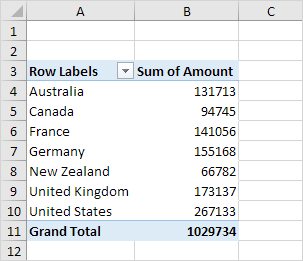### Calculated Field

A calculated field uses the values from another field. To insert a calculated field, execute the following steps.

1. Click any cell inside the pivot table.

2. On the PivotTable Analyze tab, in the Calculations group, click Fields, Items & Sets.3. Click Calculated Field.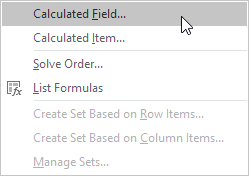The Insert Calculated Field dialog box appears.

4. Enter Tax for Name.

5. Type the formula =IF(Amount>100000, 3%*Amount, 0)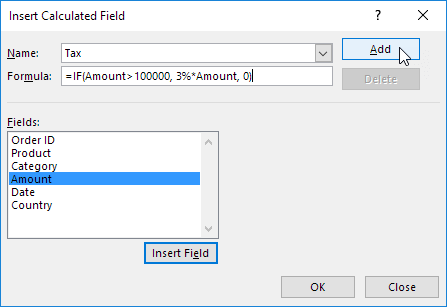Note: use the Insert Field button to quickly insert fields when you type a formula. To delete a calculated field, select the field and click Delete (under Add).

7. Click OK.

Excel automatically adds the Tax field to the Values area.

Result: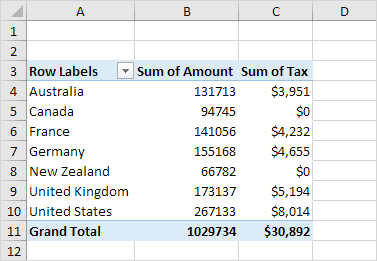### Calculated Item

A calculated item uses the values from other items. To insert a calculated item, execute the following steps.

1. Click any Country in the pivot table.

2. On the PivotTable Analyze tab, in the Calculations group, click Fields, Items & Sets.3. Click Calculated Item.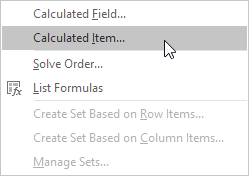The Insert Calculated Item dialog box appears.

4. Enter Oceania for Name.

5. Type the formula =3%*(Australia+'New Zealand')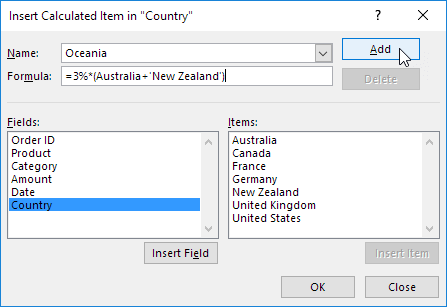Note: use the Insert Item button to quickly insert items when you type a formula. To delete a calculated item, select the item and click Delete (under Add).

7. Repeat steps 4 to 6 for North America (Canada and United States) and Europe (France, Germany and United Kingdom) with a 4% and 5% tax rate respectively.

8. Click OK.

Result: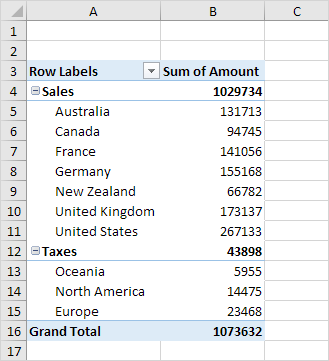Note: we created two groups (Sales and Taxes).

Go to Next Chapter: Tables